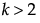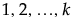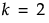Tip: When you have completed the Factors outline, consider selecting Save Factors from the red triangle menu. This saves the factor names, roles, changes, and values in a data table that you can later reload.
Factors Outline
Adds multiple factors. Enter the number of factors to add, click Add Factor, and then select the factor type. Repeat Add N Factors to add multiple factors of different types.
The name of the factor. When added, a factor is given a default name of X1, X2, and so on. To change this name, double-click it and enter the desired name.
 • To edit a factor name, double-click the factor name.
 • Categorical factors have a down arrow to the left of the factor name. Click the arrow to add a level.
 • To remove a factor level, click the value, click Delete, and click outside the text box.
 • To modify the entry under Changes, click the value in the Changes column and select the appropriate entry.
 • To edit a value, click the value in the Values column.
To choose a factor type, click Add Factor in Custom Design.
The default values for a discrete numeric factor with k levels, where, are the integers. The default values for a discrete numeric factor withlevels are -1 and 1. Replace the default values with the settings that you plan to use in your experiment.
In the assumed model, the effects for a discrete numeric factor with k levels include polynomial terms in that effect through order k-1. For k greater than 6, powers up to the 5th level are included. The Estimability for polynomial effects (powers of two or higher) is set to If Possible. This allows the algorithm to use the multiple levels as permitted by the run size. If the polynomial terms are not included, then a main effects only design is created. For more details about how discrete numeric factors are treated in the assumed model, see Model.
For designs with Hard or Very Hard to change factors, Custom Design strives to find a design that is optimal, given your specified optimality criterion. See Optimality Criteria. For details about the methodology used to generate split-plot designs, see Jones and Goos (2007). For details relating to designs with hard-to-change covariates, see Jones and Goos (2015).
Factors and Design Generation Outline for a Split-Split-Plot Design shows a split-split-plot scenario, using the factors from the Cheese Factors.jmp sample data table (located in the Design Experiment folder).
Factors and Design Generation Outline for a Split-Split-Plot Design
 • Each level of Whole Plots corresponds to a block of constant settings of the hard-to-change factors.
 •
 • The factor Whole Plots is assigned the Design Role column property with a value of Random Block.
When you designate Changes as both Hard and Very Hard, categorical factors called Subplots and Whole Plots are added to the design. This situation results in a split-split-plot design:
 • Each level of Subplots corresponds to a block of constant settings of the hard-to-change factors.
 • Each level of Whole Plots corresponds to a block of constant settings of the very-hard-to-change factors.
 • The Model script in the design table applies the Random Effect attribute to the Whole Plots and Subplots effects.
 • The levels of the hard-to-change factor are assumed to be nested within the levels of the very-hard-to-change factor by default.
 • In the design table, both of the factors Whole Plots and Subplots are assigned the Design Role column property with a value of Random Block.
To construct a two-way split-plot design, select the Hard to change factors can vary independently of Very Hard to change factors option under Design Generation. The option crosses the levels of the hard-to-change factor with the levels of the very-hard-to-change factor. See Two-Way Split-Plot Designs.

Help created on 9/19/2017